## Example Questions

### Example Question #51 : How To Find The Solution To An Equation

Consider the following equation: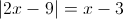Which of the following must be true?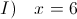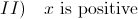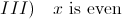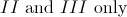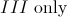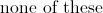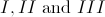Explanation:

The quantity inside the absolute value brackets must equal either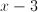or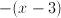, depending on whether the quantity inside the brackets is positive or negative. We therefore have two seperate equations: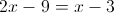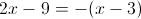To solve the first equation, add 9 to both sides:Subtractfrom both sides: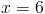This is the first solution. Now let's look at the second equation. The distributive law gives us: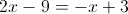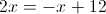Addto both sides: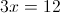Divide both sides by 3: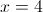Therefore,is either 4 or 6.

Statement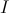does NOT have to be true becausecan also equal 4.

Statement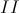must be true because both 4 and 6 are positive .

Finally, statement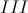always holds because 4 and 6 are both even.

### Example Question #51 : How To Find The Solution To An Equation

If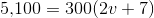,

then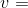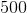Explanation:

Divide both sides by 300 to get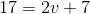.  Subtract 7 and divide by two to get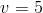.

### Example Question #1 : How To Find The Solution To An Equation

What is the value of (5 + x)(10 – y) when x = 3 and y = –3?

104

38

56

108

104

Explanation:

This is a simple plug-in and PEMDAS problem. First, plug in x = 3 and y = –3 into the x and y. You should follow the orders of operation and compute what is within the parentheses first and then find the product. This gives 8 * 13 = 104. The answer is 104.

### Example Question #2 : How To Find The Solution To An Equation

If x = 4, and y = 3x + 5, then 2y – 1 equals

33

22

15

47

33

Explanation:

Start by plugging in x = 4 to solve for y: y = 3 * 4 + 5 = 17.  Then 2 * 17 – 1 = 33

### Example Question #21 : Equations / Inequalities

Sarah’s current age is three times Ron’s age two years ago. Sarah is currently 14 years older than Ron. What is the sum of Sarah and Ron’s current age?

36

34

32

24

34

Explanation:

The best way to solve this problem is to turn the two statements into equations calling Sarah’s age S and Ron’s age R. So, S = 3(R – 2) and S = 14 + R. Now substitute the value for S in the second equation for the value of S in the first equation to get 14 + R = 3(R – 2) and solve for R. So R equals 10 so S equals 24 and the sum of 10 and 24 is 34.

### Example Question #22 : Equations / Inequalities

A store sells potatoes for $0.24 and tomatoes for$0.76. Fred bought 12 individual vegetables. If he paid \$6.52 total, how many potatoes did Fred buy?

7

2

5

8

5

Explanation:

Set up an equation to represent the total cost in cents: 24P + 76T = 652. In order to reduce the number of variables from 2 to 1, let the # tomatoes = 12 – # of potatoes. This makes the equation 24P + 76(12 – P) = 652.

Solving for P will give the answer.

### Example Question #5 : How To Find The Solution To An Equation

Kim is twice as old as Claire. Nick is 3 years older than Claire. Kim is 6 years older than Emily. Their ages combined equal 81. How old is Nick?

27

17

22

13

17

Explanation:

The goal in this problem is to have only one variable. Variable “x” can designate Claire’s age.

Then Nick is x + 3, Kim is 2x, and Emily is 2x – 6; therefore x + x + 3 + 2x + 2x – 6 = 81

Solving for x gives Claire’s age, which can be used to find Nick’s age.

### Example Question #6 : How To Find The Solution To An Equation

If 6h – 2g = 4g + 3h

In terms of g, h = ?

2g

4g

3g

5g

g

2g

Explanation:

If we solve the equation for b, we add 2g to, and subtract 3h from, both sides, leaving 3h = 6g. Solving for h we find that h = 2g.

### Example Question #7 : How To Find The Solution To An Equation

If 2x + y = 9 and y – z = 4 then 2x + z = ?

5

13

29

21

Cannot be determined

5

Explanation:

If we solve the first equation for 2x we find that 2x = 9 – y. If we solve the second equation for z we find z = –4 + y. Adding these two manipulated equations together we see (2x) + (y) = (9 – y)+(–4 + y).

The y’s cancel leaving us with an answer of 5.

### Example Question #8 : How To Find The Solution To An Equation

11/(x – 7) + 4/(7 – x) = ?

15/(x – 7)

15/(7 – x)

7/(7 – x)

15

(–7)/(7 – x)